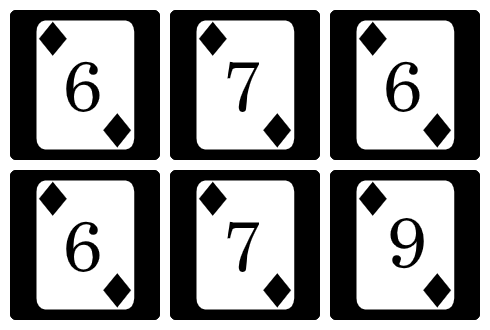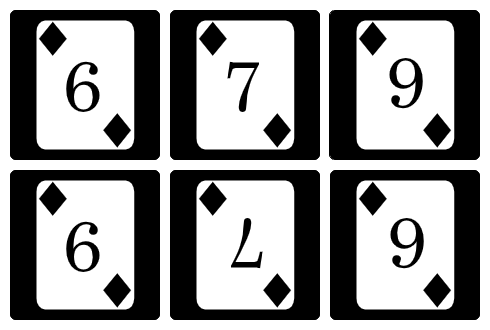시간 제한메모리 제한제출정답맞힌 사람정답 비율
1 초 (추가 시간 없음) 1024 MB31212511742.086%

## 문제

Jaehyun likes digits. Among the 10 digits, 6, 7, 8, and 9 are his favorite. Therefore, he made a special card set consisting of only 6, 7, 8 and 9.

Currently, Jaehyun has $N\times M$ cards. Jaehyun wants to make a magical $N$ by $M$ matrix of cards. Each row of the matrix should contain $M$ cards. He already arranged his cards in a shape of $N$ by $M$ matrix.Figure 1. Initial state, not point symmetric.

To be a magic matrix, the matrix must be point symmetrical: Rotating the matrix 180 degrees results in the same original matrix. For example, 8 is point symmetrical with itself, and 6 and 9 are point symmetrical with each other.

Jaehyun doesn't want to switch the position of the cards, so his goal is to make the matrix point symmetrical by only rotating the cards in their original positions.Figure 2. After rotating two cards, they are point symmetric.

Find the minimum number of cards you have to turn to make a magic matrix.

## 입력

The first line contains two integers, $N$ and $M$. ($1 \le N,\ M \le 500$)

Each of the next $N$ lines contains a string of $M$ characters which denotes the numbers written in each card. It is guaranteed that each character is one of 6, 7, 8 or 9.

## 출력

Print the minimum number of cards you have to turn to make a magic matrix in the first line. If it is not possible to make a magic matrix, print "-1". (without quotes)

## 예제 입력 1

2 3
676
679


## 예제 출력 1

2


## 예제 입력 2

3 3
888
888
888


## 예제 출력 2

0


## 예제 입력 3

1 1
7


## 예제 출력 3

-1


## 출처

• 문제를 만든 사람: ainta